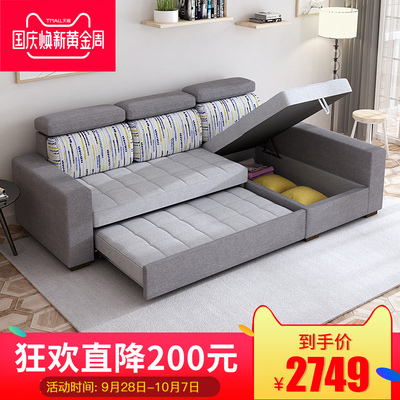﻿ 牧眠7251沙发床可折叠年中大促，牧眠布艺沙发床领取优惠券 -阅美客

# 牧眠7251沙发床可折叠年中大促• 品牌:牧眠
• 型号:7251
• 沙发骨架:金属
• 填充物:高弹泡沫海绵
• 是否可拆换:沙发套可拆换
• 风格:简约现代
• 附加功能:多功能
• 家具结构:支架结构
• 材质:其他
• 是否可定制:是
• 适用对象:成人
• 可送货/安装:北京南昌市哈尔滨市大连市沈阳市西安市贵阳市郑州市金华市长沙市常州市成都市东莞市佛山市福州市广州市杭州市南京市南宁市宁波市青岛市上海深圳市苏州市台州市天津温州市无锡市武汉市厦门市重庆
• 产地:广东省
• 地市:佛山市
• 区县:顺德区
• 出租车是否可运输:否
• 安装说明详情:无安装说明
• 是否可预售:是
• 是否组装:整装
• 款式定位:品质奢华型
• 设计元素:大师设计
• 颜色分类:2.6(双+左贵)A012.6(双+右贵)A012.6(双+左贵)A022.6(双+右贵)A022.6(双+左贵)A032.6(双+右贵)A032.6(双+左贵)A042.6(双+右贵)A042.8(双+左贵)A012.8(双+右贵)A012.8(双+左贵)A022.8(双+右贵)A022.8(双+左贵)A032.8(双+右贵)A032.8(双+左贵)A042.8(双+右贵)A043.3(双+单+贵)A013.3(双+单+贵)A023.3(双+单+贵)A033.3(双+单+贵)A043.3(双+单+踏+贵)A013.3(双+单+踏+贵)A023.3(双+单+踏+贵)A033.3(双+单+踏+贵)A04
• 包装长度:2米以上
• 包装体积:2